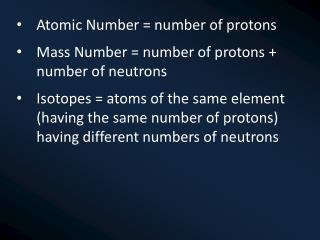DownloadDownload PresentationAtomic Number = number of protons Mass Number = number of protons + number of neutrons

# Atomic Number = number of protons Mass Number = number of protons + number of neutrons

Télécharger la présentation## Atomic Number = number of protons Mass Number = number of protons + number of neutrons

- - - - - - - - - - - - - - - - - - - - - - - - - - - E N D - - - - - - - - - - - - - - - - - - - - - - - - - - -
##### Presentation Transcript

1. Atomic Number = number of protons • Mass Number = number of protons + number of neutrons • Isotopes = atoms of the same element (having the same number of protons) having different numbers of neutrons

2. White, cloudy stuff (precipitate) forms • Precipitation reaction, because precipitate has been formed • Barium nitrate + sodium hydroxide -> barium hydroxide + sodium nitrate • Ba(NO3)2(aq) + 2NaOH(aq) -> Ba(OH)2(s) + 2NaNO3(aq) • The solubility rule states that nitrate ions always form soluble compounds so no insoluble precipitate could be formed.

3. Sodium carbonate + calcium chloride -> sodium chloride + calcium carbonate • Na2CO3(aq) + CaCl2(aq) -> 2NaCl(aq) + CaCO3(s)

4. The solution turns blue (copper ions), the copper dissolves and silvery/grey powder forms • Displacement reaction, because copper has displaced the silver ions in the solution • Copper + silver nitrate -> silver + copper nitrate • Cu(s) + 2AgNO3(aq) -> 2Ag(s) + Cu(NO3)2(aq) • Gold is less reactive than silver so is unable to replace the silver ions in the solution

5. H2 + O2 → H2O2 NaCl + AgNO3 → AgCl + NaNO3 CuSO4 + Zn → ZnSO4 + Cu NaOH + ZnSO4 → Na2SO4 + Zn(OH)2 HCl + NaOH → NaCl + H2O Mg + O2 → MgO H2 + O2 → H2O CaO + HCl → CaCl2 + H2O 2 2 2 2 2 2